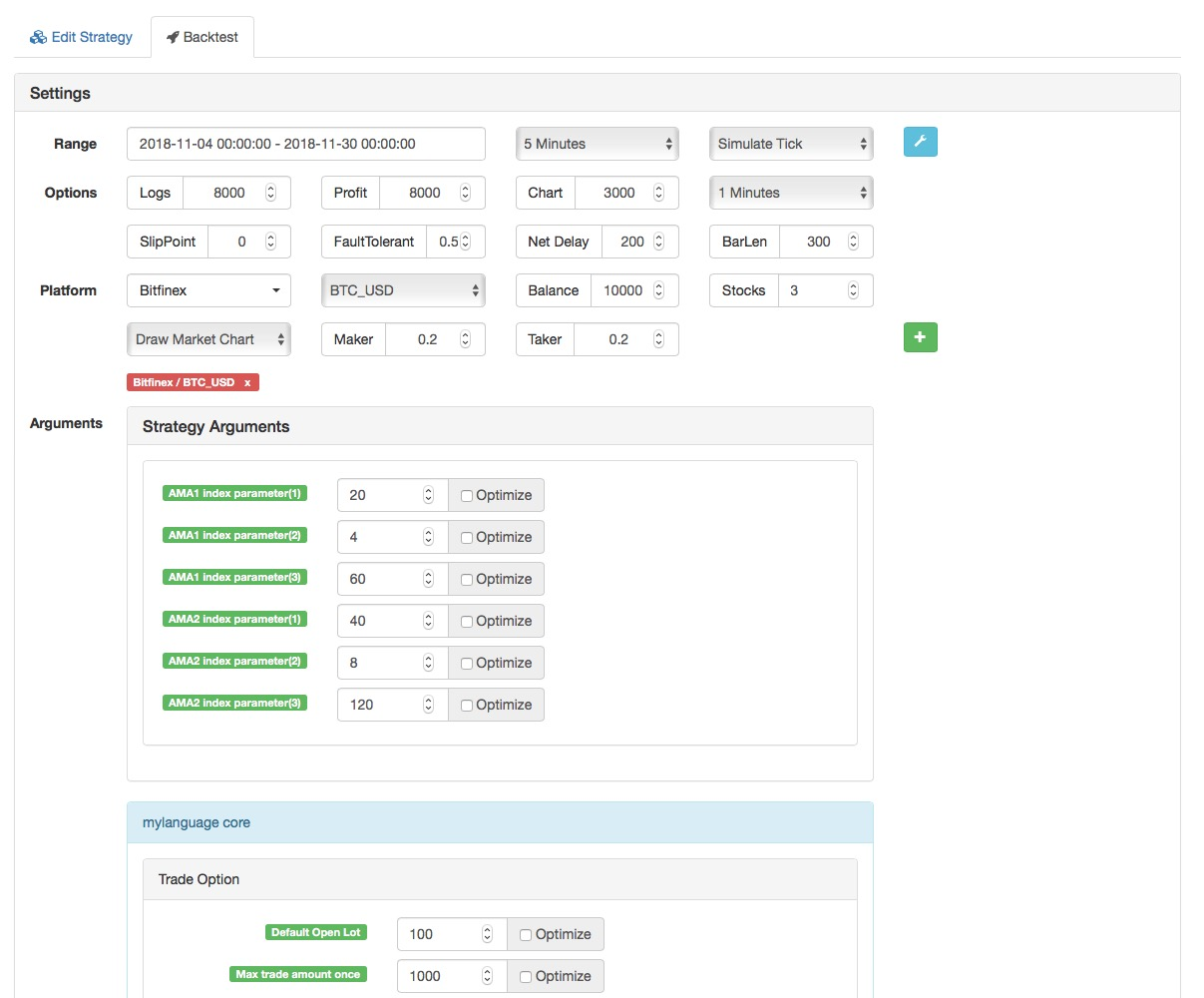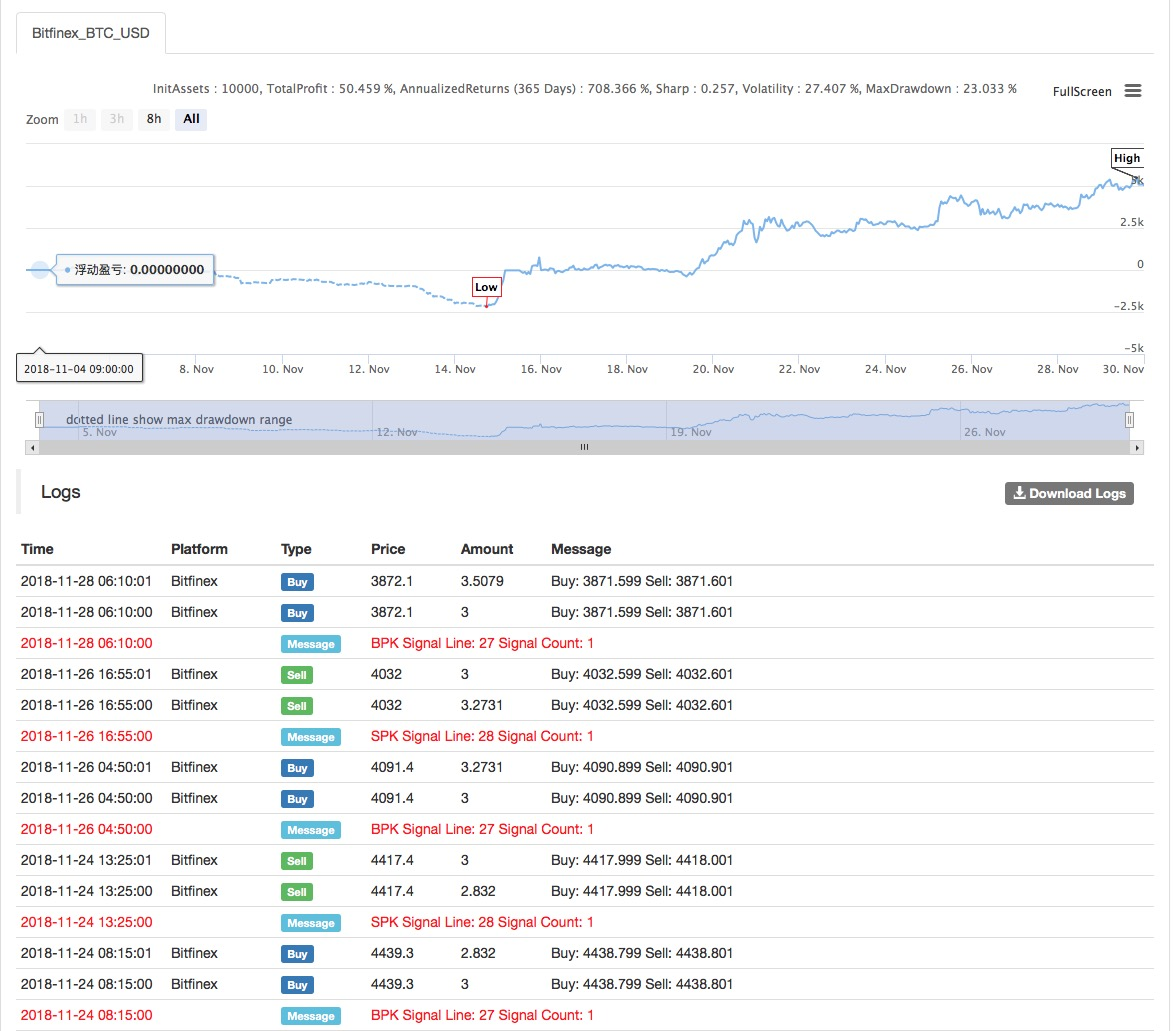# [原创] DMI指标配合阶段高低价的量化交易策略

DMI指标

DMI趋势线

DMI是给定时期内范围扩展的移动平均值（默认值为14天）。正向移动指标（+DMI）衡量价格向上移动的强度;负向移动指标（-DMI）衡量价格向下移动的强度。这两条线反映了牛与熊的相应强度。``````AMA1指标，公式：AMA1^^EMA(DMA(CLOSE,CQ1),2);
AMA2指标，公式：AMA2^^EMA(DMA(CLOSE,CQ2),2);
``````

My语言源码：

``````DIR1:=ABS(CLOSE-REF(CLOSE,N));
VIR1:=SUM(ABS(CLOSE-REF(CLOSE,1)),N);
ER1:=DIR1/VIR1;
CS1:=ER1*(2/(N1+1)-2/(N2+1))+2/(N2+1);
CQ1:=CS1*CS1;
AMA1^^EMA(DMA(CLOSE,CQ1),2);

DIR2:=ABS(CLOSE-REF(CLOSE,M));
VIR2:=SUM(ABS(CLOSE-REF(CLOSE,1)),M);
ER2:=DIR2/VIR2;
CS2:=ER2*(2/(M1+1)-2/(M2+1))+2/(M2+1);
CQ2:=CS2*CS2;
AMA2^^EMA(DMA(CLOSE,CQ2),2);

cq22:CQ2;
aa:DMA(CLOSE,CQ2);

BKVOL=0  AND  REF(AMA1,1)<REF(AMA2,1)  AND  AMA2<AMA1,BPK;
SKVOL=0  AND  REF(AMA1,1)>REF(AMA2,1)  AND  AMA2>AMA1,SPK;
``````

##### 相关下载
###### 登录后评论
Copyright © 2019 宽客在线